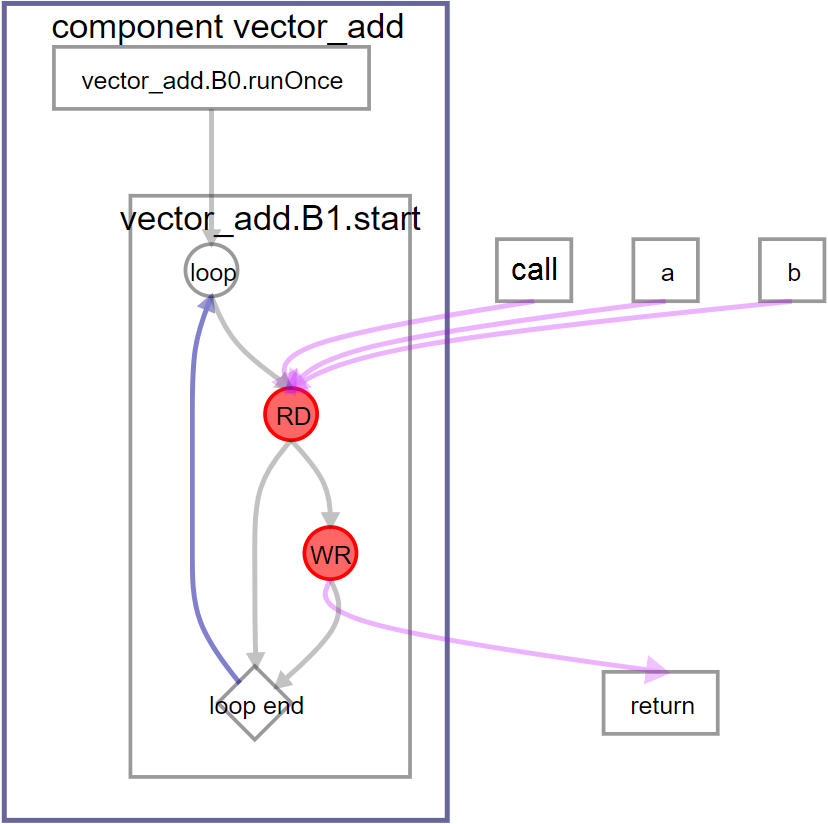ID 683152
Date 6/02/2023
Public

## 4.1.6. Pass-by-Value Interface

For software developers accustomed to writing code that targets a CPU, passing each element in an array by value might be unintuitive because it typically results in many function calls or large parameters. However, for code that targets an FPGA device, passing array elements by value can result in smaller and simpler hardware on the FPGA device.

The vector addition example can be coded to pass the vector array elements by value as follows. A struct is used to pass the entire array (of 8 data elements) by value.

When you use a struct to pass the array, you must also do the following things:
• Define element-wise copy constructors.
• Define element-wise copy assignment operators.
• Add the hls_register memory attribute to all struct members in the definition.
struct int_v8 {
hls_register int data;

//copy assignment operator
int_v8 operator=(const int_v8& org) {
#pragma unroll
for (int i=0; i< 8; i++) {
data[i]       = org.data[i]      ;
}
return *this;
}

//copy constructor
int_v8 (const int_v8& org) {
#pragma unroll
for (int i=0; i< 8; i++) {
data[i]       = org.data[i]      ;
}
}

//default construct & destructor
int_v8() {};
~int_v8() {};
};

int_v8 a,
int_v8 b) {
int_v8 c;
#pragma unroll 8
for (int i = 0; i < 8; ++i) {
c.data[i] = a.data[i]
+ b.data[i];
}
return c;
}


This component takes and processes only eight elements of vector a and vector b, and returns eight elements of vector c. To compute 1024 elements for the example, the component needs to be called 128 times (1024/8). While in previous examples the component contained loops that were pipelined, here the component is invoked many times, and each of the invocations are pipelined.

The following diagram shows the Function View in the System Viewer that is generated when you compile this example.
Figure 28. System Viewer Function View of vector_add Component with Pass-By-Value InterfaceThe latency of this component is one, and it has a loop initiation interval (II) of one.
Compiling this component with an Intel® Quartus® Prime compilation flow targeting an Intel® Arria® 10 device results in the following QoR metrics:
Table 6.  QoR Metrics Comparison for Pass-by-Value Interface1
QoR Metric Pointer Avalon® MM Host Avalon® MM Agent Avalon® ST Pass-by-Value
ALMs 15593.5 643 490.5 314.5 130
DSPs 0 0 0 0 0
RAMs 30 0 48 0 0
fMAX (MHz)2 298.6 472.37 498.26 389.71 581.06
Latency (cycles) 24071 142 139 134 128
Initiation Interval (II) (cycles) ~508 1 1 1 1
 1The compilation flow used to calculate the QoR metrics used Intel® Quartus® Prime Pro Edition Version 17.1. 2The fMAX measurement was calculated from a single seed.
The QoR metrics for the vector_add component with a pass-by-value interface shows fewer ALM used, a high component fMAX, and optimal values for latency and II. In this case, the II is the same as the component invocation interval. A new invocation of the component can be launched every clock cycle. With an initiation interval of 1, 128 component calls are processed in 128 cycles so the overall latency is 128.